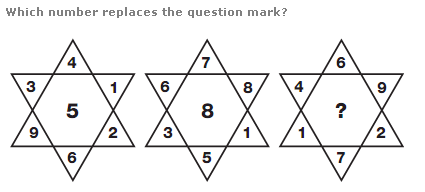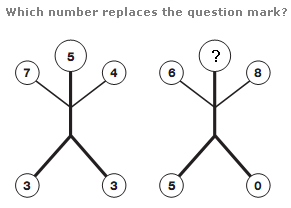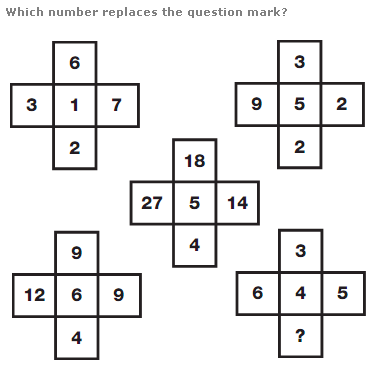# Puzzles - Number puzzles

### Exercise :: Number puzzlesAnswer : 11 Explanation : In each star, the number in the centre equals the difference between the sum of the numbers in the upwards triangle and the sum of the numbers in the downward triangle.Answer : 2 Explanation : In each diagram, multiply the numbers shown on the arms together and add the number at the very top to give a 2 digit result, written in the lower 2 spaces.Answer : 0 Explanation : Use the top two diagrams as a source. Numbers in corresponding positions in the lower left diagram equal the sums of the numbers in the top two diagrams, numbers in the central diagram equal their product, and numbers in the lower right diagram equal their difference.